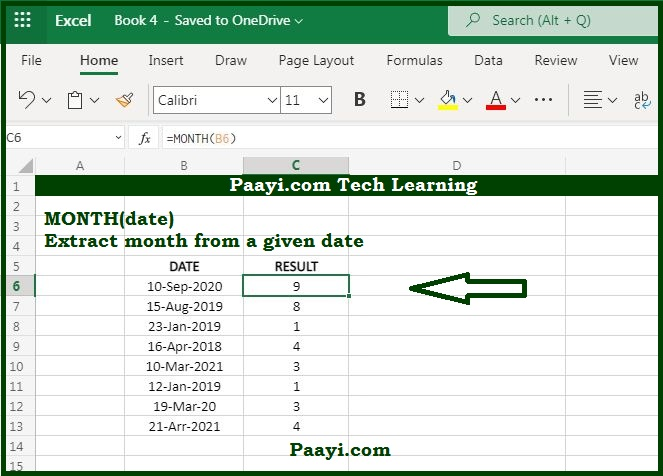# Learn How to Use Microsoft Excel MONTH Function

Written by | 0 Comments | 787 Views

In this article, you will learn how to use the Microsoft Excel MONTH function and its prime function in Microsoft Excel. You will also get to know the Microsoft Excel MONTH function return value and syntax with the help of some examples.

## Microsoft Excel MONTH Function

The purpose of the MONTH function is to get a month as a number (1-12) from a date. That means the Microsoft Excel MONTH function extracts a month from a specified date to a number from 1 to 12. So, you can use the MONTH function to extract a month number from a date into a cell. You can also feed a month number into another function similar to the DATE function.

MONTH Function Return Value

It will return the value from 1 to 12.

### MONTH Function Syntax

= MONTH(date)

Where:

• date: A valid Excel date.

## How to Use Microsoft Excel MONTH Function?As we know, the MONTH function extracts a month from a given date as a number between 1 to 12. You can understand this by the examples given below:

• = MONTH("17-Sep-2020") - This will return a value 9.
• = MONTH("26-Jan-2020") - This will return a value 1.